RRB JE EEE (CBT I) Mock Test- 10

# RRB JE EEE (CBT I) Mock Test- 10

Test Description

## 100 Questions MCQ Test RRB JE Mock Test Series Electrical & Electronics Engineering | RRB JE EEE (CBT I) Mock Test- 10

RRB JE EEE (CBT I) Mock Test- 10 for Railways 2022 is part of RRB JE Mock Test Series Electrical & Electronics Engineering preparation. The RRB JE EEE (CBT I) Mock Test- 10 questions and answers have been prepared according to the Railways exam syllabus.The RRB JE EEE (CBT I) Mock Test- 10 MCQs are made for Railways 2022 Exam. Find important definitions, questions, notes, meanings, examples, exercises, MCQs and online tests for RRB JE EEE (CBT I) Mock Test- 10 below.
Solutions of RRB JE EEE (CBT I) Mock Test- 10 questions in English are available as part of our RRB JE Mock Test Series Electrical & Electronics Engineering for Railways & RRB JE EEE (CBT I) Mock Test- 10 solutions in Hindi for RRB JE Mock Test Series Electrical & Electronics Engineering course. Download more important topics, notes, lectures and mock test series for Railways Exam by signing up for free. Attempt RRB JE EEE (CBT I) Mock Test- 10 | 100 questions in 90 minutes | Mock test for Railways preparation | Free important questions MCQ to study RRB JE Mock Test Series Electrical & Electronics Engineering for Railways Exam | Download free PDF with solutions
 1 Crore+ students have signed up on EduRev. Have you?
RRB JE EEE (CBT I) Mock Test- 10 - Question 1

### Find the minimum number of straight lines to make the given figure.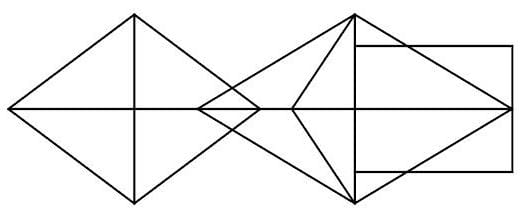Detailed Solution for RRB JE EEE (CBT I) Mock Test- 10 - Question 1 We get,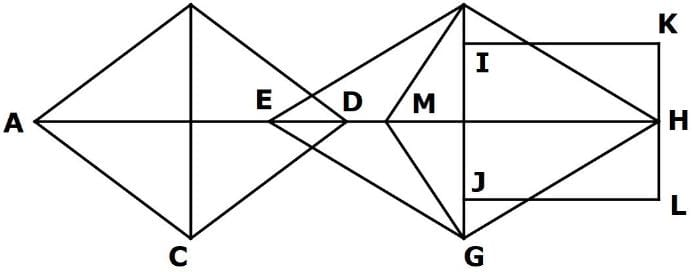Minimum required straight lines are - AB, AC, BC, AH, BD, CD, EF, EG, FH, GH, FG, FM, MG, IK, KL and LJ.

Total = 16

RRB JE EEE (CBT I) Mock Test- 10 - Question 2

### If 1.96 of 1.2 of a = 3.43 of 1.6 of b. Find b: a.

Detailed Solution for RRB JE EEE (CBT I) Mock Test- 10 - Question 2

1.96 of 1.2 of a = 3.43 of 1.6 of b

1.96 x 1.2 x a = 3.43 x 1.6 x b

3a = 7b

b: a = 3: 7

RRB JE EEE (CBT I) Mock Test- 10 - Question 3

### Following bar graph shows the sales of clothes of 3 Brands: Levi's, Lee Cooper and Spykar in Central Mall in Bangalore for the months of October, November and December.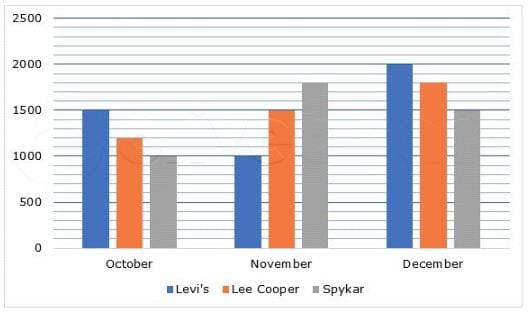For all 3 months, what is the difference between the sum of the number of Levi's and Spykar clothes sold and the number of Lee Cooper clothes sold?

Detailed Solution for RRB JE EEE (CBT I) Mock Test- 10 - Question 3 sum of the number of Levi's and Spykar clothes sold

= 1500 + 1000 + 1000 + 1800 + 2000 + 1500 = 8800

number of Lee Cooper clothes sold

1200 + 1500 + 1800 = 4500

Difference = 8800 - 4500 = 4300

RRB JE EEE (CBT I) Mock Test- 10 - Question 4

What will come in place of (?) in this equation?

? ÷ [(5/12) of (12/25) of 125 ÷ {√(21 + √16)/2}] = ? + 3

Detailed Solution for RRB JE EEE (CBT I) Mock Test- 10 - Question 4 Let ? be denoted by 'x'.

x ÷ [(5/12) of (12/25) of 125 ÷ {√(21 + √16)/2}] = x + 3

⇒ x ÷ [(5/12) of (12/25) of 125 ÷ {√(21 + 4)/2}] = x + 3

⇒ x ÷ [(5/12) of (12/25) of 125 ÷ {√(25)/2}] = x + 3

⇒ x ÷ [(5/12) of (12/25) of 125 ÷ {5/2}] = x + 3

⇒ x ÷ [25 ÷ 2.5] = x + 3

⇒ x/10 = x + 3

⇒ x = 10x + 30

⇒ x = −10/3

RRB JE EEE (CBT I) Mock Test- 10 - Question 5

How many triangles are present in the given figure?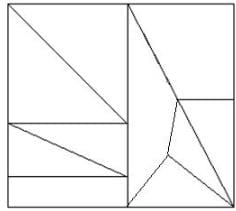Detailed Solution for RRB JE EEE (CBT I) Mock Test- 10 - Question 5

There are 9 triangles in the given figure.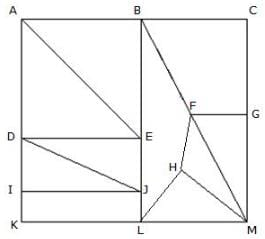Triangles are - ABE, ADE, BLM, BCM, DEJ, DJI, FHM, FGM, HLM.

RRB JE EEE (CBT I) Mock Test- 10 - Question 6

Calculate the difference between mean deviation and variance of given numbers.

3, 6, 8, 1, 4, 6, 4, 2, 2, 4

Detailed Solution for RRB JE EEE (CBT I) Mock Test- 10 - Question 6

Mean = (3 + 6 + 8 + 1 + 4 + 6 + 4 + 2 + 2 + 4)/10 = 4

Now, distance of each numbers from their mean are:

4 - 3 = 1

6 - 4 = 2

8 - 4 = 4

4 - 1 = 3

4 - 4 = 0

6 - 4 = 2

4 - 4 = 0

4 - 2 = 2

4 - 2 = 2

4 - 4 = 0

Mean deviation = (1 + 2 + 4 + 3 + 0 + 2 + 0 + 2 + 2 + 0)/10 = 1.6

Variance = (12 + 22 + 42 + 32 + 02 + 22 + 02 + 22 + 22 + 02)/10 = 4.2

Difference = 4.2 - 1.6 = 2.6

RRB JE EEE (CBT I) Mock Test- 10 - Question 7

What is the unit digit of (254+145)42?

Detailed Solution for RRB JE EEE (CBT I) Mock Test- 10 - Question 7 The concept of cyclicity is used to identify the last digit of the number.

Cyclicity chart: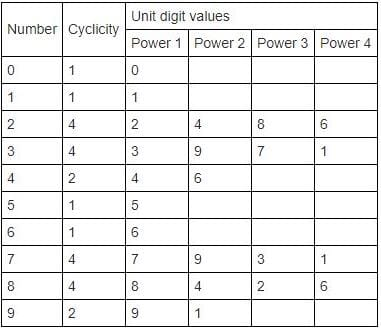(Note: when remainder is 0, power = cyclicity)

= (254+145)42

= 39942

Unit digit of 399 is 9.

From the cyclicity chart, the cyclicity of 9 is 2.

So, we have to divide the power of 399 i.e.42, by 2.

After dividing 42 by 2, remainder = 0. So, power = cyclicity = 2

Unit digit of 92=1

Hence, the unit of (254+145)42 is 1.

RRB JE EEE (CBT I) Mock Test- 10 - Question 8

What is the value of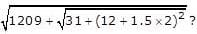Detailed Solution for RRB JE EEE (CBT I) Mock Test- 10 - Question 8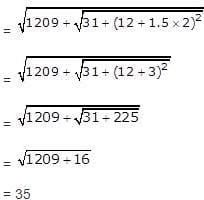RRB JE EEE (CBT I) Mock Test- 10 - Question 9

What value we get if divide (11/5) + (9/4) + (7/2) by (132 - 10)?

Detailed Solution for RRB JE EEE (CBT I) Mock Test- 10 - Question 9

(11/5) + (9/4) + (7/2)

= (44 + 45 + 70)/20

= 159/20

(132 - 10)

= 169 - 10

= 159

According to the question-

(159/20) ÷ 159

= (159/20) * (1/159)

= 1/20

= 0.05

RRB JE EEE (CBT I) Mock Test- 10 - Question 10

A Faulty watch gains 1 hour in 6 hours. After how many days will it show the correct time again?

Detailed Solution for RRB JE EEE (CBT I) Mock Test- 10 - Question 10

It will show the correct time again when it gains 24 hours.

It gains 1 hour in 6 hours

It gains 24 hours in 6*24 hours or 6 days.

RRB JE EEE (CBT I) Mock Test- 10 - Question 11

If, it is given that, 82 - 28 = 32 and 65 - 42 = 38, then, 76 - 18 = ?

Detailed Solution for RRB JE EEE (CBT I) Mock Test- 10 - Question 11 (8*2) + (2*8) = 32

(6*5) + (4*2) = 38

Similarly,

(7*6) + (1*8) = 50

RRB JE EEE (CBT I) Mock Test- 10 - Question 12

If a quadratic equation 3x2 - 4√3x + m = 0 has equal roots, then find the value of 3m2 - 5m + 16.

Detailed Solution for RRB JE EEE (CBT I) Mock Test- 10 - Question 12 Quadratic equation 3x2 - 4√3x + m = 0 has equal roots. So,

D = 0 = (-4√3)2 - 4 x 3 x m

m = 4

Therefore,

= 3m2 - 5m + 16

= 3 x 4 x 4 - 5 x 4 + 16

= 44

RRB JE EEE (CBT I) Mock Test- 10 - Question 13

How many numbers are irrational among the numbers given below?

6th root of 2187, 5th root of 4096, √(28 x 23 - 283), √(34 x 35 - 23 x 22), √4802

Detailed Solution for RRB JE EEE (CBT I) Mock Test- 10 - Question 13

1. 6th root of 2187 = 3 x 6th root of 3 (irrational)

2. 5th root of 4096 = 4 x 5th root of 4 (irrational)

3. √(28 x 23 - 283) = 19

4. √(34 x 35 - 23 x 22) = 6√19 (irrational)

5. √4802 = 49√2 (irrational)

RRB JE EEE (CBT I) Mock Test- 10 - Question 14

Select the odd one from the following options.

Detailed Solution for RRB JE EEE (CBT I) Mock Test- 10 - Question 14 Option (1)

= Product of (0.003 x 25 + 4.65) and (0.05 x 24 - 0.2)

= 4.725 x 1

= 4.725

Option (2):

= Sum of (23 x 0.005 + 4 x 0.003) and 3.598

= 0.127 + 3.598

= 3.725

Option (4):

= 2 x 3 x 5.6 x 0.005 + 45.57 x 0.1

= 4.725

Hence, option (2) is odd among all four options.

RRB JE EEE (CBT I) Mock Test- 10 - Question 15

A contractor undertakes to complete a work in 19 weeks. He employs 180 men for 7 weeks and they complete 1/2 of the work. He then reduces the number of men to 140, who work for 5 weeks. How many men must be employed for the remaining period to finish the work?

Detailed Solution for RRB JE EEE (CBT I) Mock Test- 10 - Question 15 Work done by 180 men in 7 weeks = 1260 men weeks.

This is half the work. It means the remaining half is 1260 men weeks.

Work done by 140 men in 5 weeks = 700 men weeks.

So remaining work = 1260 - 700 = 560 men weeks which has to be completed over 7 weeks.

Required number of men = 560/7 = 80

RRB JE EEE (CBT I) Mock Test- 10 - Question 16

A man bought six different fruits, and, each fruit's quantity bought is different. Quantity of Papaya bought is less than that of Oranges as well as Mango. Quantity of Apples bought is less than both Kiwi and Banana. Quantity of Mango is more than only one fruits and that of oranges is more than at least two fruits. Apple's quantity is more than that of oranges. Which fruit's quantity is less than that of three fruits?

Detailed Solution for RRB JE EEE (CBT I) Mock Test- 10 - Question 16

Quantity of Papaya bought is less than that of Oranges as well as Mango.

We get,

Case I:

Papaya < Oranges < Mango

Case II:

Papaya < Mango < Oranges

Now,

Quantity of Apples bought is less than both Kiwi and Banana.

Apple's quantity is more than that of oranges.

We get,

Oranges < Apples < Kiwi/banana < Kiwi/Banana

Since,

Quantity of Mango is more than only one fruits.

And, that of oranges is more than at least two fruits.

Thus, case I becomes invalid.

We get,

Papaya < Mango < Oranges < Apples < Kiwi/banana < Kiwi/Banana

So, Orange's quantity is less than that of three fruits.

RRB JE EEE (CBT I) Mock Test- 10 - Question 17

In ∆ABC, P and Q are points on AB and AC respectively such that AP:PB = 2:1 and AQ:QC = 2:1. If BC = 18 cm, what is the length of PQ?

Detailed Solution for RRB JE EEE (CBT I) Mock Test- 10 - Question 17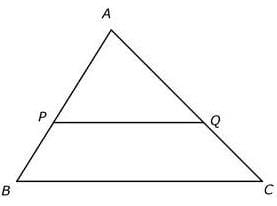Since, P and Q divide AB and AC respectively in the same ratio,

PQ is parallel to BC

In ∆APQ and ∆ABC,

∠APQ = ∠ABC and ∠AQP = ∠ACB [Corresponding angles]

So, ∆APQ and ∆ABC are similar,

Corresponding sides are proportional,

BC/PQ = AB/AP

⇒ 18/PQ = (AP + PB)/AP

⇒ 18/PQ = 1 + PB/AP

⇒ 18/PQ = 1 + ½

⇒ PQ = 18*(2/3) = 12 cm

RRB JE EEE (CBT I) Mock Test- 10 - Question 18

The Pie-Chart shows the percentage distribution of the number of employees that work in a particular department within a Company.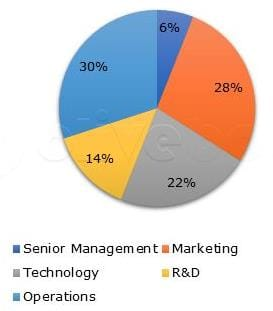If the number of employees that work in R&D is 588, what is the number of employees who work in Senior Management?

Detailed Solution for RRB JE EEE (CBT I) Mock Test- 10 - Question 18 14% of total employees = 588

Total employees = 588*(100/14) = 4200

Number of employees who work in Senior Management

= 6% of 4200 = 252

RRB JE EEE (CBT I) Mock Test- 10 - Question 19

Area of a circle is 706.5 cm2 and its radius is increased by a certain amount so that its circumference is increased by 31.4 cm. What is the new area of the circle? (Use π = 3.14)

Detailed Solution for RRB JE EEE (CBT I) Mock Test- 10 - Question 19 Let the original radius of the circle be R

Area of circle = πR2

R2 =706.5/3.14 = 225

R = 15 cm.

Let radius is increased by S cm.

Increase in circumference = (2π x (15 +S)) - (2π x15)

= 2πS

2πS = 31.4

S = 5 cm

New area of circle = π x (20)2 = 3.14 x 400 = 1256 cm2.

RRB JE EEE (CBT I) Mock Test- 10 - Question 20

What is the angle between the initial and final position of the second hand of o watch when it has travelled from 02:37:42 p.m. to 02:39:54 p.m.?

Detailed Solution for RRB JE EEE (CBT I) Mock Test- 10 - Question 20 In 15 seconds, the seconds hand travels 90°

In 1 second, the second hand travels 6°

Time duration betweenthe 02:37:42 p.m. and 02:39:54 p.m.

= 2 minutes 12 seconds.

In every minute, the second hand comes back to its original position.

Angle between initial and final position of second hand

= 12*6 = 72°

RRB JE EEE (CBT I) Mock Test- 10 - Question 21

A person has total of Rs.60000 with him, out which 60% he invested in scheme A at 15% SI for 4 years and remaining in scheme B at 25% SI for 4 years. Now, total interest after 4 years he invested in scheme B for 2 years and total principle amount he invested in scheme A for 2 years. What will be the difference between total amount of interest from both the schemes after 6 years?

Given below are the steps involved. Arrange them in the sequential order.

(A) Amount invested in scheme A and scheme B for the second time is Rs.60000 and [21600 + 24000 = Rs.45600] respectively.

(B) Required difference = (21600 + 18000) ~ (24000 + 22800) = Rs.7200

(C) Initial amount invested in scheme A and B is 60% of 60000 = Rs.36000 and 40% of 60000 = Rs.24000 respectively.

(D) Interest from scheme A and scheme B after 2 years = [(60000 * 15 * 2)/100 = Rs.18000] and [(45600 * 25 * 2)/100 = Rs.22800] respectively.

(E) Interest from scheme A and scheme B after 4 years = [(36000 * 15 * 4)/100 = Rs.21600] and [(24000 * 25 * 4)/100 = Rs.24000] respectively.

Detailed Solution for RRB JE EEE (CBT I) Mock Test- 10 - Question 21

(C) Initial amount invested in scheme A and B is 60% of 60000 = Rs.36000 and 40% of 60000 = Rs.24000 respectively.

(E) Interest from scheme A and scheme B after 4 years = [(36000 * 15 * 4)/100 = Rs.21600] and [(24000 * 25 * 4)/100 = Rs.24000] respectively.

(A) Amount invested in scheme A and scheme B for the second time is Rs.60000 and [21600 + 24000 = Rs.45600] respectively.

(D) Interest from scheme A and scheme B after 2 years = [(60000 * 15 * 2)/100 = Rs.18000] and [(45600 * 25 * 2)/100 = Rs.22800] respectively.

(B) Required difference = (21600 + 18000) ~ (24000 + 22800) = Rs.7200

RRB JE EEE (CBT I) Mock Test- 10 - Question 22

Express 0.112112112.. into (p/q) form.

Detailed Solution for RRB JE EEE (CBT I) Mock Test- 10 - Question 22

Let X = 0.112112112.. (I)

⇒ 1000X = 112.112112112.. (II)

(II) - (I) gives,

999X = 112

⇒ X = 112/999

RRB JE EEE (CBT I) Mock Test- 10 - Question 23

If 70% of (a - b) = 40% of (a + b), find a:b

Detailed Solution for RRB JE EEE (CBT I) Mock Test- 10 - Question 23 70% of (a - b) = 40% of (a + b)

⇒ 70/100 x (a - b) = 40/100 x (a + b)

⇒ 7a - 7b = 4a + 4b

⇒ 7a - 4a = 4b + 7b

⇒ 3a = 11b

⇒ a/b = 11/3

⇒ a:b = 11:3

RRB JE EEE (CBT I) Mock Test- 10 - Question 24

A is as much younger than B as he is older than C. If the sum of the ages of B and C is 15 years, what is definitely the difference between B and A's age?

Detailed Solution for RRB JE EEE (CBT I) Mock Test- 10 - Question 24 B - A = B - C => A = C.

Also, B + C = 15 => B + A = 15

So, (B - A) cannot be determined.

RRB JE EEE (CBT I) Mock Test- 10 - Question 25

What is the value of (2/3) of (6/7) of (14/15) of [63 ÷ {√625 − √(80 ÷ √(100 ÷ 8 X 2))}]?

Detailed Solution for RRB JE EEE (CBT I) Mock Test- 10 - Question 25

(2/3) of (6/7) of (14/15) of [63 ÷ {√625 − √(80 ÷ √(100 ÷ 8 X 2))}]

= (2/3) of (6/7) of (14/15) of [63 ÷ {√625 − √(80 ÷ √(12.5 X 2))}]

= (2/3) of (6/7) of (14/15) of [63 ÷ {√625 − √(80 ÷ √(25))}]

= (2/3) of (6/7) of (14/15) of [63 ÷ {√625 − √(80 ÷ 5)}]

= (2/3) of (6/7) of (14/15) of [63 ÷ {√625 − √16}]

= (2/3) of (6/7) of (14/15) of [63 ÷ {25 − 4}]

= (2/3) of (6/7) of (14/15) of [63 ÷ 21]

= (2/3) X (6/7) X (14/15) X 3

= 8/5

RRB JE EEE (CBT I) Mock Test- 10 - Question 26

The product of three numbers a, b and 21 is 10290 and their HCF is 7. If a is greater than b and b is greater than 10, then find the LCM of greatest and smallest number.

Detailed Solution for RRB JE EEE (CBT I) Mock Test- 10 - Question 26

let a = 7m , b = 7n

According to the question:

10290 = 7m x 7n x 21

10 = m x n

There are two possible values for m and n.

Case 1: when, m = 10, n = 1

a = 7m = 7 x 10 = 70

b = 7n = 7 x 1 = 7 < 10

So, this case is not valid.

Case 2: when, m = 5, n = 2

a = 7m = 7 x 5 = 35

b = 7n = 7 x 2 = 14

LCM of 35 and 14 = 70

RRB JE EEE (CBT I) Mock Test- 10 - Question 27

Find the value of 13 + (1/3) of 81 - (1/4) of 192 + 23 of 16 ÷ 92.

Detailed Solution for RRB JE EEE (CBT I) Mock Test- 10 - Question 27 = 13 + (1/3) of 81 - (1/4) of 192 + 23 of 16 ÷ 92

= 13 + 27 - 48 + 4

= - 4

RRB JE EEE (CBT I) Mock Test- 10 - Question 28

By selling 45 sweets for Rs 40 a man losses 20%. How much should he sell for Rs 24 to gain 20%?

Detailed Solution for RRB JE EEE (CBT I) Mock Test- 10 - Question 28

SP of 45 sweets = Rs 40

SP of 1 sweets = 40/45

L = 20%

CP = 100/80 * 40/45 = 10/9

SP2 = (100 + 20) / 100 * CP

SP2 = 120/100 * 10/9 = 4/3

SP of Rs 4/3 sweets for 1 sweets

SP of Rs 24 for = 24/ (4/3) = (24 * 3) / 4 = 18 sweets

RRB JE EEE (CBT I) Mock Test- 10 - Question 29

A shopkeeper has 5 items such that their mean weight is 30 kg. If weight of these items are consecutive multiples of 5, what will be the sum of the weight of the lightest and heaviest item?

Detailed Solution for RRB JE EEE (CBT I) Mock Test- 10 - Question 29 Let 'a' kg is the weight of the lightest item.

Then, the arrangement of weights in ascending order are:

a, a + 5, a + 10, a + 15, a + 20

Mean = 30

5 x 30 = a + a + 5 + a + 10 + a + 15 + a + 20

a = 20 kg

Weight of heaviest item = 20 + 20 = 40 kg

Sum of weight of lightest and heaviest item = 20 + 40 = 60 kg

RRB JE EEE (CBT I) Mock Test- 10 - Question 30

Ram had Rs 2000, part of which he lent at 15% for two years at simple interest and the rest at 10% at compound interest for two years. The total interest was Rs 528. Find the sum lent at simple interest (in Rs)?

Detailed Solution for RRB JE EEE (CBT I) Mock Test- 10 - Question 30 Rate of simple interest = 15%

Let the amount lent at SI be Rs x.

For 2 years, SI = x*15*2/100 = 30*x/100

Amount lent at CI = 2000 - x

For 2 years, CI = (2000 - x)*(1 + 10/100)2 - (2000 - x) = (2000 - x)(21/100)

30x/100 + (2000-x)*21/100 = 528

After solving we get x = Rs 1200

RRB JE EEE (CBT I) Mock Test- 10 - Question 31

In the following question, select the related letter/word/number from the given alternatives.

MUSIC : TUNE

Detailed Solution for RRB JE EEE (CBT I) Mock Test- 10 - Question 31 As tune is in music; rhythm is in dance.
RRB JE EEE (CBT I) Mock Test- 10 - Question 32

Find out similar set of the given set:

(64, 216, 125)

Detailed Solution for RRB JE EEE (CBT I) Mock Test- 10 - Question 32 The given question set (64, 216, 125) is the perfect cube of

(4, 6, 5). Similarly (8, 27, 64) is the perfect cube of (2, 3, 4).

RRB JE EEE (CBT I) Mock Test- 10 - Question 33

Pointing to a boy Neha said, ‘He is the son of my grandfather’s only child. How is she related to that boy?

Detailed Solution for RRB JE EEE (CBT I) Mock Test- 10 - Question 33 Grandfather's child is father and son of a girl's father will be her brother.
RRB JE EEE (CBT I) Mock Test- 10 - Question 34

In a code language ‘S1357’ means ‘SWe are very happy’, ‘S2639’ means ‘Sthey are extremely lucky’ , and ‘S794’ means ‘SHappy and lucky’. Which digit in that code language stands for ‘Svery’?

Detailed Solution for RRB JE EEE (CBT I) Mock Test- 10 - Question 34

01357 → we are very happy .......(i)

2639 → they are extremely lucky ......(ii)

794 → happy and lucky ......(iii)

from (i) and (ii)

3 → are

from (ii) and (iii)

9 → lucky

from (i) and (iii)

7 → happy

from (iii)

4 → and

from (i)

1, 5 → we, very

Hence, we cannot find exact code for 'very'.

RRB JE EEE (CBT I) Mock Test- 10 - Question 35

In a certain code language the word ‘FUTILE’ is written as ‘HYVMNI’. How will the word ‘PENCIL’ be written in that language?

Detailed Solution for RRB JE EEE (CBT I) Mock Test- 10 - Question 35 Odd-placed letters are coded as two places forward and even-placed letters are coded as four places forward as in English alphabet.
RRB JE EEE (CBT I) Mock Test- 10 - Question 36

Laxman start walking from his house and went 15 km to North then he turned West and covered 10 kms. Then he turned South and covered 5 kms. Finally turning to East he covered 10 kms. In which direction he is from his house?

Detailed Solution for RRB JE EEE (CBT I) Mock Test- 10 - Question 36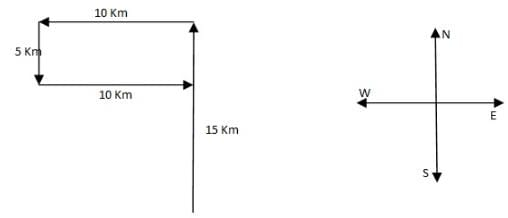From the figure its clear that laxman is in the north of his house.

RRB JE EEE (CBT I) Mock Test- 10 - Question 37

Given below are two Matrices of Twenty-five Cells, each containing two classes of alphabets. The columns and rows of Matrix I are numbered form 0 to 4 and that of Matrix II from 5 to 9. A letter from these Matrices can be represented first by its row number and next by its column number. In each of the following questions, identify one set of number pairs out of (1), (2), (3) and (4) which represents the given word.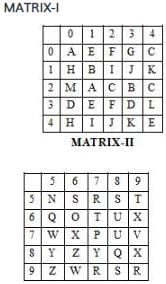RUST

Detailed Solution for RRB JE EEE (CBT I) Mock Test- 10 - Question 37

RUST

R– 57, 97, 99

U– 68, 78

S– 56, 58, 98

T– 59, 67

Therefore, the correct answer is option (3) i.e. 97, 68, 56, 59

RRB JE EEE (CBT I) Mock Test- 10 - Question 38

Arrange the following in a meaningful order:

1. Curd

2. Grass

3. Butter

4. Milk

5. Cow

RRB JE EEE (CBT I) Mock Test- 10 - Question 39

In the questions given below, some relationship has been expressed through symbols as shown below. Based on the meaning of these symbols and choose the correct answer.

ϕ means ‘less than’

Δ means ‘not greater than’

- means ‘equal to’

+ means ‘not equal to’

× means ‘not less than’

= means ‘greater than’

X ϕ Y - Z implies

Detailed Solution for RRB JE EEE (CBT I) Mock Test- 10 - Question 39

Clearly, the meaning of the given symbols

X ϕ Y - Z ⇒ X < Y = Z

Using the proper notations/symbols in option (c), we get

X + Y × Z ⇒ X ≠ Y > Z ⇒ X < Y = Z

Therefore, X ϕ Y - Z ⇒ X + Y × Z.

RRB JE EEE (CBT I) Mock Test- 10 - Question 40

Choose the odd one out from the given alternatives.

Detailed Solution for RRB JE EEE (CBT I) Mock Test- 10 - Question 40

Except MOTHER: TMPRHD, the rest of the group, follow the arrangement given below.

Pattern is-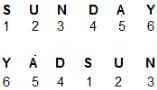RRB JE EEE (CBT I) Mock Test- 10 - Question 41

Find the odd one out from the given alternatives.

Detailed Solution for RRB JE EEE (CBT I) Mock Test- 10 - Question 41

Except in pair 33- 56, in all others the sum of digits of one number is equal to the sum of digits of all the second number.

RRB JE EEE (CBT I) Mock Test- 10 - Question 42

In the question below, there are few statements followed by few conclusions. You have to take the given statements to be true even if they seem to be at variance with commonly known facts and then decide which of the given conclusion logically follow(s) from the given statements.

Statements:

All horses are rivers.

All rivers are jungles.

No Jungle is flower.

Conclusions:

I. No horse is flower.

II. No flower is horse.

Detailed Solution for RRB JE EEE (CBT I) Mock Test- 10 - Question 42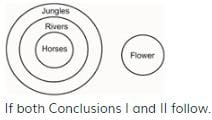RRB JE EEE (CBT I) Mock Test- 10 - Question 43

Choose the missing terms out of the given alternatives.

Ef_h_gfe_hef_ehgefg_

Detailed Solution for RRB JE EEE (CBT I) Mock Test- 10 - Question 43 The given cyclic order series follows the pattern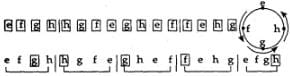RRB JE EEE (CBT I) Mock Test- 10 - Question 44

What should come next in the following letter sequence:

A A B A B C A B C D A B C D E A B C D E F A B C D E F G A B C D E F G

Detailed Solution for RRB JE EEE (CBT I) Mock Test- 10 - Question 44 The sequence is A AB ABC ABCD.........
RRB JE EEE (CBT I) Mock Test- 10 - Question 45

In the given figure, circle represents youths, the triangle represents the persons who are in job and rectangle represents illiterate person.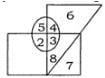Which of the following number depicts, illiterate youths who have a job?

Detailed Solution for RRB JE EEE (CBT I) Mock Test- 10 - Question 45 The number of illiterate youths and who have a job are three.
RRB JE EEE (CBT I) Mock Test- 10 - Question 46

What comes in place of the question markthe ?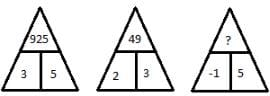Detailed Solution for RRB JE EEE (CBT I) Mock Test- 10 - Question 46 The numbers obtained by squaring the numbers at the bottom are combined together physically to get the number in the upper part.
RRB JE EEE (CBT I) Mock Test- 10 - Question 47

what comes in place of ?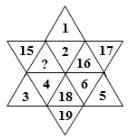Detailed Solution for RRB JE EEE (CBT I) Mock Test- 10 - Question 47 The given figure contains numbers 1 to 6 in three alternate segments, the smaller number being towards the outside and the numbers 14 to 19 in the remaining three alternate segments with the smaller number towards the inside.
RRB JE EEE (CBT I) Mock Test- 10 - Question 48

Find the water image of the object given in the question figure.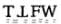Detailed Solution for RRB JE EEE (CBT I) Mock Test- 10 - Question 48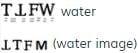RRB JE EEE (CBT I) Mock Test- 10 - Question 49

A Piece of paper is folded and cut as shown below in the question fiqure. From the given answer fiqures, indicate how it will appear when opened.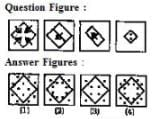Detailed Solution for RRB JE EEE (CBT I) Mock Test- 10 - Question 49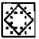RRB JE EEE (CBT I) Mock Test- 10 - Question 50

In the following questions, four/five figures are given. Three/four are similar in a certain way and so form a group. Find out which one of the figures does not belong to that group.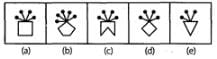Detailed Solution for RRB JE EEE (CBT I) Mock Test- 10 - Question 50 In all other figures, the number of pins at the top of the figure are equal to the number of sides of the figure.
RRB JE EEE (CBT I) Mock Test- 10 - Question 51

Which of the following words cannot be formed from 'GENERALIZATION'?

Detailed Solution for RRB JE EEE (CBT I) Mock Test- 10 - Question 51 Using the letters of the given word, the word 'LIZARD' cannot be formed.
RRB JE EEE (CBT I) Mock Test- 10 - Question 52

If ‘+’ stands for multiplication, ‘x’ stands for division, ‘–’ stands for addition and ‘÷’ stands for subtraction, what is the answer for the following equation?

20 – 5 ÷ 18 x (3 + 2) = ?

Detailed Solution for RRB JE EEE (CBT I) Mock Test- 10 - Question 52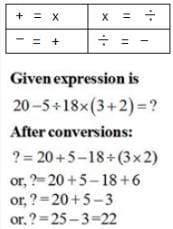RRB JE EEE (CBT I) Mock Test- 10 - Question 53

The day on 18.09.1977 was Sunday. A couple was married on this date. In the next 15 years, there how many marriage anniversaries would fall on Sunday?

Detailed Solution for RRB JE EEE (CBT I) Mock Test- 10 - Question 53 1977 is an ordinary year. We know that the calendar of an ordinary year repeats after 6 yrs or 11 yrs.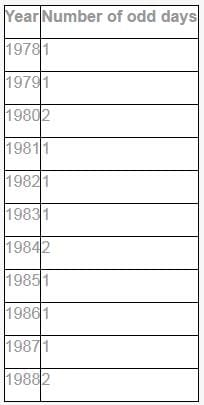Therefore,

Number of odd days from 18.09.1977 to 18.09.1983=7, i.e., 0 odd day

It means that in 1983, 18th September would fall on Sunday.

and number of odd days from 18.09.1977 to 18.09.1988 = 14, i.e., 0 odd day.

Now, it is clear that 2 marriage anniversaries would fall on Sunday in the next 15 yr.

RRB JE EEE (CBT I) Mock Test- 10 - Question 54

In a row of girls, if Rohini who is 12th from the left and Mihika who is 9th from the right, if they interchange their seats then Rohini becomes 18th from the left. How many girls are there in the row?

Detailed Solution for RRB JE EEE (CBT I) Mock Test- 10 - Question 54 When Rohini and Mihika interchange their places, then Rohini becomes on 18th place, it means Rohini who was on the 12th place comes at the 6th place after crossing 5 places. Therefore, there are 5 girls between Rohini and Mihika. So, the total number of the girls in the row = (18 + 9) -1 = 26 or (15 + 12) – 1 = 26.
RRB JE EEE (CBT I) Mock Test- 10 - Question 55

Study the diagram given below and answer the question.

Which part represents those clerks who are graduates and have government job?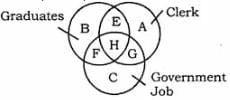Detailed Solution for RRB JE EEE (CBT I) Mock Test- 10 - Question 55 'H' represents those clerks who are graduates and have government job.
RRB JE EEE (CBT I) Mock Test- 10 - Question 56

Who was recently elected as the Deputy Chairman of the Rajya Sabha in the Parliament?

Detailed Solution for RRB JE EEE (CBT I) Mock Test- 10 - Question 56 Harivansh Narayan Singh Rajya Sabha MP from Bihar since 2014 was recently elected as the new Deputy Chairman of Rajya Sabha in the Parliament.

Earlier in his career he was a journalist with 'Prabhat Khabar' and then was chosen as the media advisor to former Prime Minister Chandra Shekhar.

RRB JE EEE (CBT I) Mock Test- 10 - Question 57

Recently, the Ministry of External Affairs organised 11th World Hindi Conference at which of the following Indian ocean states?

Detailed Solution for RRB JE EEE (CBT I) Mock Test- 10 - Question 57 11th edition of World Hindi Conference was organised in Swami Vivekanand International Convention Centre, Pailles Mauritius.

Theme of the Conference: "Hindi Vishwa Aur Bharatiy Sanskriti". It was organised by the Ministry of External Affairs, Government of India in association with the Government of Mauritius

RRB JE EEE (CBT I) Mock Test- 10 - Question 58

Right to Information, an important legislative act to promote transparency & strengthen the democracy of India came into being on which year?

Detailed Solution for RRB JE EEE (CBT I) Mock Test- 10 - Question 58 On October 12, 2005, Right To Information came into being after passage of an Act in the Indian parliament to bring in transparency in governmental procedures and end corruption

Using rtionline.gov.in citizens of India can seek information to turn India into a participatory democracy.

According to the government guidelines, 'Any person who desires to obtain information shall submit a written or electronic request in English or Hindi or in the official language of the area to the Central Public Information Officer or his/her counterpart at the state level.'

RRB JE EEE (CBT I) Mock Test- 10 - Question 59

Which Indian state excelled to be the top performer in the recently released 'Ease of Living Index 2018' by Ministry of Housing & Urban Affairs?

Detailed Solution for RRB JE EEE (CBT I) Mock Test- 10 - Question 59 Andhra Pradesh topped the charts among Indian States in terms of "Ease of Living Index 2018" rankings launched by the Ministry of Housing and Urban Affairs, followed by Odisha and Madhya Pradesh.

The Index seeks to assist states in undertaking a 360-degree assessment of their strengths, weaknesses, opportunities, and threat & innovate to perform in the governance, identity and culture, education, health, safety and security, economy, affordable housing, land use planning etc. for its residents.

RRB JE EEE (CBT I) Mock Test- 10 - Question 60

Which of the following government entity started 'Seva Bhoj Yojna' - a scheme to reimburse central share of CGST and IGST on food, prasad, langar etc. offered by religious and charitable institutions?

Detailed Solution for RRB JE EEE (CBT I) Mock Test- 10 - Question 60 Ministry of culture recently launched the Seva Bhoj Yojna, a scheme to reimburse the central share of CGST and IGST on food, prasad, langar, etc. offered by religious and charitable institutions.

Institutions like Temples, Gurudwara, Mosque, Church, Dharmik Ashram, Dargah etc. who are established and serving free food for more than 5 years to more than 5000 people every month without any discrimination are the eligible entities.

RRB JE EEE (CBT I) Mock Test- 10 - Question 61

Google recently, unveiled ____________ which will quickly render any PDF with Indian language content into editable text using Artificial Intelligence.

Detailed Solution for RRB JE EEE (CBT I) Mock Test- 10 - Question 61 During the 4th edition of 'Google for India' event, the Project Navlekha was unveiled which in Sanskrit means "a new way to write". It will quickly render any PDF with Indian language content into editable text using Artificial Intelligence.

Under the project, The registered publishers will be provided with free web hosting service along with a branded domain for 3 years and having AdSense support to help monetising their content.

RRB JE EEE (CBT I) Mock Test- 10 - Question 62

Which of the following Indian states launched a scheme, 'Kanyashree', to incentivize adolescent girl child education to combat issue of child marriages?

Detailed Solution for RRB JE EEE (CBT I) Mock Test- 10 - Question 62 Kanyashree was launched in 2013 by the West Bengal government to prevent child marriage in economically backward sections with an annual household income of Rs.1.2 lakh.

Eligible girls got Rs.1,000 a year scholarship from ages 13 to 18 years and a one-time grant of Rs.25,000 on remaining unmarried till 18 years. More than 5 million girl children benefitted from the scheme.

Recently the annual income limit was relaxed to any girl child within the age limit.

RRB JE EEE (CBT I) Mock Test- 10 - Question 63

Which of the following government institution launched "Pitch to MOVE", a business opportunity platform for budding entrepreneurs?

Detailed Solution for RRB JE EEE (CBT I) Mock Test- 10 - Question 63 NITI Aayog recently launched "Pitch to MOVE", a business opportunity platform for budding entrepreneurs to promote their business ideas.

It aims to identify and incentivise the start-ups, which will help the Government realize its vision of Shared, Connected, Intermodal and Environment Friendly Mobility for India.

The objective is to harness the latest disruption for generating employment and growth in our country

RRB JE EEE (CBT I) Mock Test- 10 - Question 64

Ghoomar is a popular traditional folk dance from which region in India?

Detailed Solution for RRB JE EEE (CBT I) Mock Test- 10 - Question 64 Ghoomar is a traditional dance from Rajasthan's Bhil tribe performed only by the women during celebrations pertaining to Goddess Saraswathi, Diwali, Holi etc.

Attire for women: Ghagharas along with colourful chunaris and heavy embroidery. Works garment.

RRB JE EEE (CBT I) Mock Test- 10 - Question 65

Which of the following International Organisations are headquartered in Washington D.C., USA?

Detailed Solution for RRB JE EEE (CBT I) Mock Test- 10 - Question 65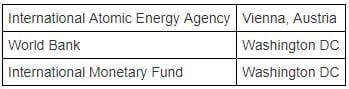RRB JE EEE (CBT I) Mock Test- 10 - Question 66

Match the following trophies with their respective sports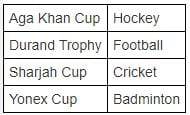Detailed Solution for RRB JE EEE (CBT I) Mock Test- 10 - Question 66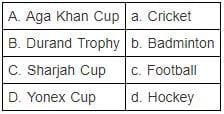RRB JE EEE (CBT I) Mock Test- 10 - Question 67

Dhauladhar National Park is situated in which of the following Indian state?

Detailed Solution for RRB JE EEE (CBT I) Mock Test- 10 - Question 67 Dhauladhar National Park is situated at a distance of 13kms from the city of Palampur in the Indian state of Himachal Pradesh.It is filled with exotic flora and fauna, and is home to different types of deer, red fox, sambhar, Asiatic lion, angora rabbits, black bears and leopards.It covers an area of 30 acres.
RRB JE EEE (CBT I) Mock Test- 10 - Question 68

Daman & Diu is Union territory of India comprising 2 different areas separated by which of the following?

Detailed Solution for RRB JE EEE (CBT I) Mock Test- 10 - Question 68

Arabian Sea separates the two areas of the union territory, Daman & Diu, bordered by the state of Gujarat.

With an area of only 112 square kms, it is the second smallest UT of India after Lakshadweep.

RRB JE EEE (CBT I) Mock Test- 10 - Question 69

Recently, BOMBAY NATURAL HISTORY SOCIETY opened its regional centre at which of the following important Wetland in India?

Detailed Solution for RRB JE EEE (CBT I) Mock Test- 10 - Question 69 Recently, BOMBAY NATURAL HISTORY SOCIETY opened its regional centre in the premises of The Wetland Research and Training Centre (WRTC) of the Chilika Development Authority (CDA) at Chandrapur in Chilika.

Here, BNHS would be engaged in identifying the air route of the migratory birds flocking the Chilika lake during winter & sample collection, training related to bird census among other important conservatory purposes.

RRB JE EEE (CBT I) Mock Test- 10 - Question 70

With which of the following Financial Institution would you associate 'Prompt Corrective Action'?

Detailed Solution for RRB JE EEE (CBT I) Mock Test- 10 - Question 70 The Reserve Bank of India has specified certain regulatory guidelines, as a part of prompt corrective action (PCA) Framework, in terms of 3 parameters,

- capital to risk weighted assets ratio (CRAR),

- net non-performing assets (NPA) and

- Return on Assets (RoA), applicable only to commercial banks and not extended to co-operative banks, non-banking financial companies (NBFCs) & other financial institutes

RRB JE EEE (CBT I) Mock Test- 10 - Question 71

Which of the following rays exhibits the property of light wave?

Detailed Solution for RRB JE EEE (CBT I) Mock Test- 10 - Question 71 Gamma rays, X-rays, microwaves and radio waves behave as a light wave and they exhibit properties such as reflection, refraction, diffraction etc.
RRB JE EEE (CBT I) Mock Test- 10 - Question 72

A ball thrown vertically upwards with an initial velocity of 19.6 m/s returns in 4 seconds. Which one of the following is the maximum height attained by the ball?

Detailed Solution for RRB JE EEE (CBT I) Mock Test- 10 - Question 72 Time taken by ball to the highest point = 4/2

= 2 Second

Now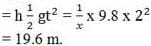RRB JE EEE (CBT I) Mock Test- 10 - Question 73

Which amongst the following is the most elastic?

Detailed Solution for RRB JE EEE (CBT I) Mock Test- 10 - Question 73 Steel is the most elastic
RRB JE EEE (CBT I) Mock Test- 10 - Question 74

A transformer works with

Detailed Solution for RRB JE EEE (CBT I) Mock Test- 10 - Question 74 A transformer works with Alternating current.
RRB JE EEE (CBT I) Mock Test- 10 - Question 75

The material having maximum ductility is:

Detailed Solution for RRB JE EEE (CBT I) Mock Test- 10 - Question 75 The material having maximum ductility is Mild steel
RRB JE EEE (CBT I) Mock Test- 10 - Question 76

Magnetic effect of current was discovered by

Detailed Solution for RRB JE EEE (CBT I) Mock Test- 10 - Question 76 Magnetic effect of current was discovered by Oersted.
RRB JE EEE (CBT I) Mock Test- 10 - Question 77

Modern communication systems use -

Detailed Solution for RRB JE EEE (CBT I) Mock Test- 10 - Question 77 Modern communication systems use Digital circuits
RRB JE EEE (CBT I) Mock Test- 10 - Question 78

Madam Curie invented Radium. She was born in ……….....

Detailed Solution for RRB JE EEE (CBT I) Mock Test- 10 - Question 78 Madam Curie was born in Poland.
RRB JE EEE (CBT I) Mock Test- 10 - Question 79

When a body is immersed in a liquid, the force acting on it is?

Detailed Solution for RRB JE EEE (CBT I) Mock Test- 10 - Question 79 According to the Principle of Flotation, when a body is immersed in a fluid, two forces act on it: (1) The weight of the body acting vertically downward through the centre of gravity of the body, and (2) The upthrust of the fluid acting vertically upward through the centre of gravity of the fluid displaced, i.e.. centre of buoyancy. The upthrust is also termed as buoyant force.
RRB JE EEE (CBT I) Mock Test- 10 - Question 80

Which of the following is the best conductor of Electricity ?

Detailed Solution for RRB JE EEE (CBT I) Mock Test- 10 - Question 80 This is because salt water is a good conductor of electricity. Salt molecules are made of sodium ions and chlorine ions. (An ion is an atom that has an electrical charge because it has either gained or lost an electron.)
RRB JE EEE (CBT I) Mock Test- 10 - Question 81

Mach number is used in connection with the speed of -

Detailed Solution for RRB JE EEE (CBT I) Mock Test- 10 - Question 81 Mach number is used in connection with the speed of Aircraft.
RRB JE EEE (CBT I) Mock Test- 10 - Question 82

Most soluble in water is —

Detailed Solution for RRB JE EEE (CBT I) Mock Test- 10 - Question 82 Water is polar so any compound that is polar is soluble in water. Sugar (C12H22O11) is a polar covalent molecule. When it dissolves in water, the weak bonds between the carbon, hydrogen and oxygen atoms are broken.
RRB JE EEE (CBT I) Mock Test- 10 - Question 83

If linear momentum is increased by 50%, the kinetic energy will increase by

Detailed Solution for RRB JE EEE (CBT I) Mock Test- 10 - Question 83 If linear momentum is increased by 50%, the kinetic energy will increase by 125%
RRB JE EEE (CBT I) Mock Test- 10 - Question 84

Which of the following is not a semiconductor?

Detailed Solution for RRB JE EEE (CBT I) Mock Test- 10 - Question 84 Arsenic is not a semiconductor.
RRB JE EEE (CBT I) Mock Test- 10 - Question 85

Which is critically endangered and species

RRB JE EEE (CBT I) Mock Test- 10 - Question 86

Which of the following colour will scatter the most while passing through a prism?

Detailed Solution for RRB JE EEE (CBT I) Mock Test- 10 - Question 86 Lights of different colour depending upon their scattering in ascending ordor are violet, indigo, blue, green, yellow, orange and red.
RRB JE EEE (CBT I) Mock Test- 10 - Question 87

Brass gets discoloured in air due to the presence of which gas in air ?

Detailed Solution for RRB JE EEE (CBT I) Mock Test- 10 - Question 87 Hydrogen sulphide can corrode metals such as iron, steel, copper, and brass, tarnish silverware, discolour copper and brass utensils, cause yellow or black stains on fixtures and alter the appearance and taste of cooked foods.
RRB JE EEE (CBT I) Mock Test- 10 - Question 88

Bleaching powder is made from —

Detailed Solution for RRB JE EEE (CBT I) Mock Test- 10 - Question 88 A mixture with lime and calcium chloride, it is marketed as chlorine powder or bleaching powder for water treatment and as a bleaching agent.
RRB JE EEE (CBT I) Mock Test- 10 - Question 89

At high altitudes the boiling point of water reduces because -

Detailed Solution for RRB JE EEE (CBT I) Mock Test- 10 - Question 89 As we go to higher altitudes the atmospheric pressure reduces, which actually lowers the boiling point of water.
RRB JE EEE (CBT I) Mock Test- 10 - Question 90

The point, through which the whole weight of the body acts, irrespective of its position, is known as

Detailed Solution for RRB JE EEE (CBT I) Mock Test- 10 - Question 90 The point, through which the whole weight of the body acts, irrespective of its position, is known as centre of gravity
RRB JE EEE (CBT I) Mock Test- 10 - Question 91

Vitamin A is also known as ?

Detailed Solution for RRB JE EEE (CBT I) Mock Test- 10 - Question 91 Retinol: Retinol is an active form of vitamin A. It produces the pigments in the retina of the eye.
RRB JE EEE (CBT I) Mock Test- 10 - Question 92

Avogadro constant, NA is the number of molecules in one mole of a substance and its value (NA) is

Detailed Solution for RRB JE EEE (CBT I) Mock Test- 10 - Question 92 Avogadro constant, NA is the number of molecules in one mole of a substance and its value (NA) is

6.02 × 1023 mol

RRB JE EEE (CBT I) Mock Test- 10 - Question 93

Kaziranga National Park is located in state of

RRB JE EEE (CBT I) Mock Test- 10 - Question 94

Ginger is an example of –

Detailed Solution for RRB JE EEE (CBT I) Mock Test- 10 - Question 94 Ginger is a rhizome (modified plant stem).
RRB JE EEE (CBT I) Mock Test- 10 - Question 95

When CaC2 is heated in atmospheric nitrogen in an electric furnance the compound formed is ________

Detailed Solution for RRB JE EEE (CBT I) Mock Test- 10 - Question 95 Solubility of alkali metal hydroxide increases as the size of the alkali metal increases.
RRB JE EEE (CBT I) Mock Test- 10 - Question 96

Brass is an alloy of —

Detailed Solution for RRB JE EEE (CBT I) Mock Test- 10 - Question 96 Zinc and Copper: Brass is a metallic alloy that is made of copper and zinc.
RRB JE EEE (CBT I) Mock Test- 10 - Question 97

Dioptre is the unit of:

Detailed Solution for RRB JE EEE (CBT I) Mock Test- 10 - Question 97 Dioptre is the unit of Power of a lens.
RRB JE EEE (CBT I) Mock Test- 10 - Question 98

In which of the following blocks of the periodic table are the Alkali & Alkaline earth metals included?

Detailed Solution for RRB JE EEE (CBT I) Mock Test- 10 - Question 98 The alkali and alkaline earth metals are included in the s-block of the periodic table.
RRB JE EEE (CBT I) Mock Test- 10 - Question 99

Cockroach is a:

Detailed Solution for RRB JE EEE (CBT I) Mock Test- 10 - Question 99 Cockroaches are omnivores and can eat almost any type of organic material in order to survive, which makes them one of the healthiest insects on the planet. Although they prefer sweets, meats and starches, they are also known to consume other items such as hair, books and decaying matter.
RRB JE EEE (CBT I) Mock Test- 10 - Question 100

Permanent hardness of water may be removed by the addition of ____

Detailed Solution for RRB JE EEE (CBT I) Mock Test- 10 - Question 100 Sodium carbonate, Na2CO3, is also known as washing soda. It can remove temporary and permanent hardness from water.

## RRB JE Mock Test Series Electrical & Electronics Engineering

11 tests
 Use Code STAYHOME200 and get INR 200 additional OFF Use Coupon Code
Information about RRB JE EEE (CBT I) Mock Test- 10 Page
In this test you can find the Exam questions for RRB JE EEE (CBT I) Mock Test- 10 solved & explained in the simplest way possible. Besides giving Questions and answers for RRB JE EEE (CBT I) Mock Test- 10, EduRev gives you an ample number of Online tests for practice

11 tests